# Long Division Steps: Lesson for Kids

Lesson Transcript
Instructor: T.J. Hoogsteen

T.J. is currently a grade 5 teacher and Vice-Principal. He has a master's degree in Educational Administration and is working toward an Ed.D. in Educational Leadership.

This lesson shows the process for completing long division equations, which involves division, multiplication, and subtraction. We'll look at equations with and without remainders and equations. Updated: 01/17/2020

## What Is Long Division?

Alex was in the middle of building a small house and he lost track of time. Suddenly, it was getting dark. With no shelter, he was afraid of wild animals and other intruders. But he did have one hope: he had a pile of bricks (56 blocks, to be exact) and in a last-ditch effort, he was going to build a shelter.

To do it, Alex would need to take the 56 blocks and turn them into four walls. How would he figure out how many blocks would go into each wall? He would use long division, the method of finding the answer to complex division problems by writing out each step of the process.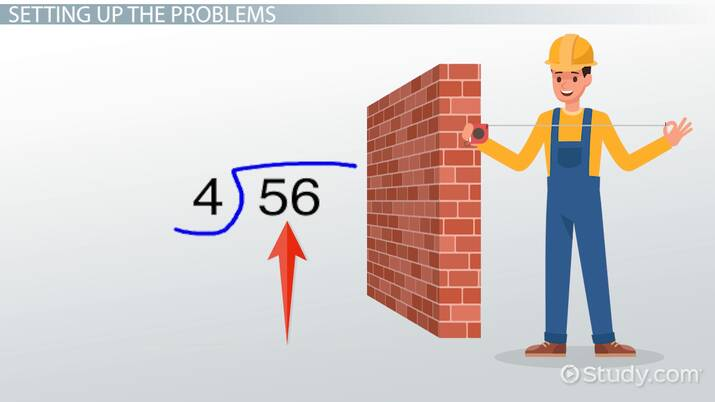An error occurred trying to load this video.

Try refreshing the page, or contact customer support.

Coming up next: Estimation: Lesson for Kids

### You're on a roll. Keep up the good work!

Replay
Your next lesson will play in 10 seconds
• 0:04 What Is Long Division?
• 0:36 Setting Up the Problems
• 1:02 Solving
• 2:20 Remainders
• 3:13 Lesson Summary
Save Save

Want to watch this again later?

Timeline
Autoplay
Autoplay
Speed Speed

## Setting Up the Problems

When using long division, we write out equations using a long division bracket. The dividend (the number being divided) goes inside the bracket, while the divisor (the number by which the dividend is being divided) goes to the left of the bracket. Alex's problem would look like this, with the dividend (56) inside the brackets and the divisor (4) outside the bracket :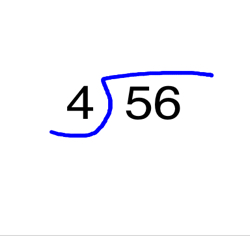Once you begin to solve the problem, the quotient, the answer to the division problem, will be placed on top of the bracket.

## Solving

There are four main steps to solving a long division problem:

1. Divide
2. Multiply
3. Subtract
4. Drop down the last digit

Let's work through these steps with Alex's long division problem.

#### 1. Divide

Start by looking at the digit that's in the largest place value of the dividend, which is always the first digit (in this case, it's 5). You'll find how many times the divisor goes into the largest place digit. So, in this case, you need to find how many times 4 goes into 5. The quotient is 1, and that goes above the bracket over the first digit of the dividend: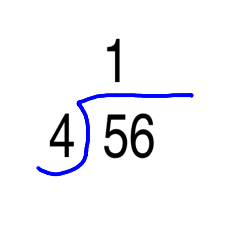#### 2. Multiply

Next, multiply the divisor outside of the bracket by the quotient on top of the bracket. In Alex's case, that would be 4 x 1, which equals 4. Place the answer (4) beneath the first digit of the dividend: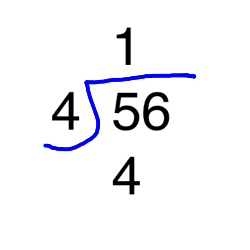#### 3. Subtract

Now, subtract the multiplication answer found in the last step from the first digit of the dividend. In this case, it would be 5 - 4, which equals 1.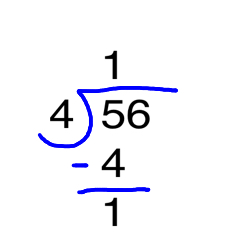#### 5. Drop down the next digit

So far, only one digit in the dividend has been divided, so the other digit still needs to be divided. To do that, bring it down and add it to the leftover 1: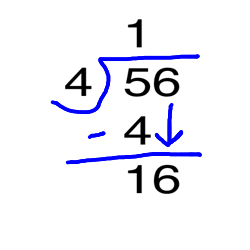To unlock this lesson you must be a Study.com Member.

### Register to view this lesson

Are you a student or a teacher?